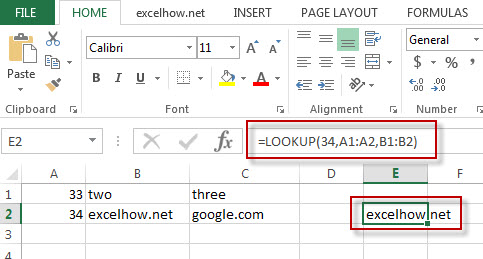Excel Lookup Function

This post will guide you how to use Excel LOOKUP function with syntax and examples in Microsoft excel.

Description

The Excel LOOKUP function will search a value in a vector or array.

The LOOKUP function is a build-in function in Microsoft Excel and it is categorized as a Lookup and Reference Function.

The LOOKUP function is available in Excel 2016, Excel 2013, Excel 2010, Excel 2007, Excel 2003, Excel XP, Excel 2000, Excel 2011 for Mac.

Syntax

The syntax of the LOOKUP function is as below:

= LOOKUP (lookup_value, lookup_vector, [result_vector])

Where the LOOKUP function arguments are:
Lookup_value -This is a required argument.  A value that you want to search in the lookup_vector.
Lookup_vector – This is a required argument.  A excel Range that only contains one row or one column.
Result_vector – This is an optional argument. A excel Range that only contains one row or one column. It must be the same size as Lookup_vector. The lookup function will look up the value in the lookup_value range and returns the value from the same position in the result_vector.
Note: if Result_vector is omitted, the Lookup function will return the first column data.

Example

The below examples will show you how to use Excel LOOKUP Lookup and Reference Function to search a value from a vector or array.

#1 To look up the value “34”in  A1:A2 range, then get the value from the same row or column in B1:B2 range, just using the following excel formula: =LOOKUP(“34”,A1:A2,B1:B2)More Excel LOOKUP Formula Examples

• Finding the Max and Min value in an Alphanumeric Data
if you want to find the Maximal or minimal string value from an alphanumeric data list in the range B1:B5, you can create a formula based on the LOOKUP function and the COUNTIF function.….
• Convert Weekday Name to Number
Assuming that you have list of data B1:B7 that contain weekday names, you want to convert all weekday names to numbers.You can use a formula based on the MATCH function to achieve the result
• List all Worksheet Names
Assuming that you have a workbook that has hundreds of worksheets and you want to get a list of all the worksheet names in the current workbook. And the below will introduce 3 methods with you..…
• Copy and Paste Only Non-blank Cells
If you want only copy non-blank cells in a range in Excel, you need to select the non-blank cells firstly, then press Ctrl +C keys to copy the selected cells. So how to only select all non-blank cells in the selected range in your worksheet..…
Related Posts

Average the Last N Numeric Values in Excel

AVERAGE function is one of the most popular functions in Excel. Apply AVERAGE together with some other functions, we can calculate average simply for some complex situations. In this article, we will introduce you to calculate average of the last ...

How to Find the First or Last Positive or Negative Number in a Column/List in Excel

Suppose we have a list of data entry, we want to look up the first positive number among them, is there any way to find it out? And if we want to look up the first or last negative number, ...

How to Find the Last or First Value in a Range Greater Than X in Excel

This post will guide you how to find the first value greater than a specified value in a range of cells in Excel. How do I find the last value greater that a given value in a row with a ...

How to Find the First or Last Non-blank Cell in a Row or Column in Excel

This post will guide you how to find the first non-blank cell in a row or column or a range in Excel. How do I get the value of the last non-blank cell in a row or column using formula ...

How to VLOOKUP to Return Value if Date Falls between Two dates in Excel

This post will guide you how to do a VLOOKUP between two dates and return corresponding value in Excel. How do I VLOOKUP between two dates and return corresponding value with lookup formula in Excel. How to lookup a value ...

Copy and Paste Only Non-blank Cells

This post will guide you how to copy and paste only non-blank cells in Excel 2013/2016. How do I copy only non blank cells with VBA code in Excel. Or how to get non-blank cells from a range of cells ...

List all Worksheet Names

This post will guide you how to get a list of all worksheet names in an excel workbook. How do I List the Sheet names with Formula in Excel. How to generate a list of all sheet tab names using ...

Convert Weekday Name to Number

This post will guide you how to convert weekday string to number in excel. How do I convert weekday name to number with a formula in excel. How to convert day of week to number in excel. Is there an ...

Finding the Max and Min value in an Alphanumeric Data

This post will guide you how to find the maximal and minimal value or string from an alphanumeric data list in excel. How do I retrieve or get the max or min string value based on alphabetic order from a ...

How to get last match that cell contains one of several values in a range

This post explains that how to check a cell that contains one of several values in another range, and then return the last match value.  And we talked that how to test a cell to see that if it contains ...

Sidebar